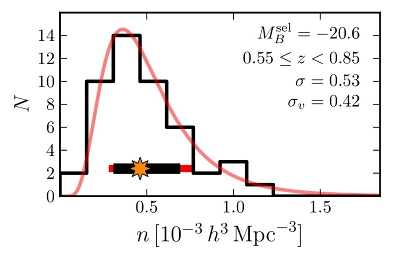## Cosmic variance

One fundamental uncertainty in any observational measurement derived from galaxy surveys is the relative cosmic variance (σv, also called sample variance), arising from the underlying large-scale density fluctuations and leading to variances larger than those expected from simple Poisson statistics. The most efficient way to tackle the cosmic variance is to split the survey into several independent areas on the sky. This minimises the sampling problem and it is better than increasing the volume in a wide contiguous field (e.g. Driver & Robotham 2010).

We take advantage of the 48 ALHAMBRA sub-fields of ~182 arcmin2 to study the cosmic variance. We measure the cosmic variance of several galaxy populations as the intrinsic dispersion in the number density distribution derived from the 48 ALHAMBRA sub-fields. We compare the observational σv with the cosmic variance of the dark matter expected from the theory, σv,dm. This provides an estimation of the galaxy bias b. We find that the galaxy bias from the cosmic variance is in excellent agreement with the bias estimated by two-point correlation function analysis in ALHAMBRA. This holds for different redshift bins, for red and blue sub-samples, and for several luminosity selections. Our results demonstrate that the cosmic variance measured in ALHAMBRA is due to the clustering of galaxies and can be used to characterise the σv affecting pencil-beam surveys. In addition, it can also be used to estimate the galaxy bias b from a method independent of correlation functions.Figure 1 - The number density distribution of MB <= -20.6 galaxies derived from the 48 ALHAMBRA sub-fields. The star and the black bar mark the median and the intrinsic dispersion (cosmic variance) of the best-fitting log-normal distribution. The red lines show the total distribution affected by Poisson errors.

Thanks to the ALHAMBRA results, we conclude that the best way to deal with the J-PAS data is to split the survey on several patches (e.g. 170 sub-fields of 50deg2 each), then study the median and the dispersion of the desired properties. This provides unbiased estimations and the extra information encoded in the intrinsic dispersion of the data.